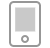# math是什么意思_math翻译_读音_用法_翻译

2019-03-05 15:44:06  ·  词库

## math是什么意思_math翻译_读音_用法_翻译

vipkid提供math是什么意思、math英语单词推荐、math英语解释、math相关词、math英语短语

【中文释义】

n. 数学（等于mathematics）;

【英文释义】
Noun:

1. a science (or group of related sciences) dealing with the logic of quantity and shape and arrangement

【math相关词】
Klamath n. 克拉马斯人[语]，克拉马斯河（美国俄勒冈州南部）;
aftermath n. 后果;余波;再生草;
mathematician n. 数学家;
mathematics n. 数学;〈诗〉同“ripen”;算学;
maths n. 数学;=mathematics（英）;
polymath n. 博学者，博识者;

【math相关词条】
math coprocessor 算术协同处理器;
math processor 数学处理机;
math processing [计] 数学处理;
math library [计] 数学程序库，数学文献库;
Math 2-plus 马赫数大于2;
binary math 二进位算术;
latter math 后割草;
math co-processor v. 数学协处理器;
silage after math 再生草;
basic math steps [计] 基本数学步骤;

## 版权声明

（1）被诉侵权的内容或文章的链接；

（2）您对该等内容或文章享有版权的证明资料；

（3）您的联系方式。我站会在接受到您的通知邮件后十四个工作日予以答复和处理。